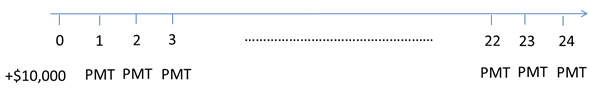# Using a Timeline to Solve Time Value of Money Problems

When solving a time value of money problem, it is sometimes easy to draw a timeline to present the cash flows on it. Once we have the timeline, we can easily understand the variables and visualize the present value or future value calculations.

In the previous pages, we demonstrated the time line for an ordinary annuity and for uneven cash flows.

Let’s take one more example to demonstrate the use of a time line.

Example: Loan Payments

You have taken a loan of \$10,000 at an annual interest rate of 12% for a period of 2 years. Calculate the monthly payments you will make on this loan.

The payments (PMT) or Equated Monthly Instalments will be paid monthly for the next 24 months.

The above problem can be demonstrated on a timeline as follows:Calculator Usage To calculate the monthly payment:Set compounding frequency to 12 (P/Y)PV = 10,000I/Y = 12N = 24PMT = 470.735